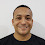### Silence Removal and End Point Detection JAVA Code

For the purpose of silence removal of captured sound, we used the algorithm  in our final year project. In this post, I am publishing the endpoint detection and silence removal code ( implementation of this algorithm in JAVA).

These links might be useful to you as well.
The constructor of following java class EndPointDetection takes two parameters
1. array of original signal's amplitude data : float[] originalSignal
2. sampling rate of original signal in Hz : int samplingRate
The Java Code :
``````package org.ioe.tprsa.audio.preProcessings;
/**
* @author Ganesh Tiwari
* @reference 'A New Silence Removal and Endpoint Detection Algorithm
* for Speech and Speaker Recognition Applications' by IIT, Khragpur
*/
public class EndPointDetection {
private float[] originalSignal; //input
private float[] silenceRemovedSignal;//output
private int samplingRate;
private int firstSamples;
private int samplePerFrame;
public EndPointDetection(float[] originalSignal, int samplingRate) {
this.originalSignal = originalSignal;
this.samplingRate = samplingRate;
samplePerFrame = this.samplingRate / 1000;
firstSamples = samplePerFrame * 200;// according to formula
}
public float[] doEndPointDetection() {
// for identifying each sample whether it is voiced or unvoiced
float[] voiced = new float[originalSignal.length];
float sum = 0;
double sd = 0.0;
double m = 0.0;
// 1. calculation of mean
for (int i = 0; i < firstSamples; i++) {
sum += originalSignal[i];
}
m = sum / firstSamples;// mean
sum = 0;// reuse var for S.D.

// 2. calculation of Standard Deviation
for (int i = 0; i < firstSamples; i++) {
sum += Math.pow((originalSignal[i] - m), 2);
}
sd = Math.sqrt(sum / firstSamples);
// 3. identifying one-dimensional Mahalanobis distance function
// i.e. |x-u|/s greater than ####3 or not,
for (int i = 0; i < originalSignal.length; i++) {
if ((Math.abs(originalSignal[i] - m) / sd) > 0.3) { //0.3 =THRESHOLD.. adjust value yourself
voiced[i] = 1;
} else {
voiced[i] = 0;
}
}
// 4. calculation of voiced and unvoiced signals
// mark each frame to be voiced or unvoiced frame
int frameCount = 0;
int usefulFramesCount = 1;
int count_voiced = 0;
int count_unvoiced = 0;
int voicedFrame[] = new int[originalSignal.length / samplePerFrame];
// the following calculation truncates the remainder
int loopCount = originalSignal.length - (originalSignal.length % samplePerFrame);
for (int i = 0; i < loopCount; i += samplePerFrame) {
count_voiced = 0;
count_unvoiced = 0;
for (int j = i; j < i + samplePerFrame; j++) {
if (voiced[j] == 1) {
count_voiced++;
} else {
count_unvoiced++;
}
}
if (count_voiced > count_unvoiced) {
usefulFramesCount++;
voicedFrame[frameCount++] = 1;
} else {
voicedFrame[frameCount++] = 0;
}
}
// 5. silence removal
silenceRemovedSignal = new float[usefulFramesCount * samplePerFrame];
int k = 0;
for (int i = 0; i < frameCount; i++) {
if (voicedFrame[i] == 1) {
for (int j = i * samplePerFrame; j < i * samplePerFrame + samplePerFrame; j++) {
silenceRemovedSignal[k++] = originalSignal[j];
}
}
}
// end
return silenceRemovedSignal;
}
}``````
The MATLAB implementation of this algorithm is also available.

1.This is a great help! Thanks soo much!!

2.can i get "c" code for this end point detection ...
if you have plz post it.....plz....

1.No, I don't have. As this code contains only the basic (linear structure) operations, it would not be difficult to code this into C. Please try yourself.

3.Hi ganesh, So Is impossible listen the voice after normalizePCM and endpointdetection?

1.you can play the recorded audio after doing those time domain operations.
you need to play the pcm array using the code : http://ganeshtiwaridotcomdotnp.blogspot.com/2011/12/java-audio-playing-pcm-amplitude-array.html

you can find other codes related to sound processing in java here :
http://ganeshtiwaridotcomdotnp.blogspot.com/search/label/Audio%20Processing

4.Hey ganesh..i am not able to listen the silence removed signal.please help me with it.as the doEndPointDetection() method returns float array and with the help of PCM code we play a byte array.please help as early as possible.

5.Hi Ganesh. can we detect starting point of the speech and if there is any silence before we start recording sound can we trim that silence so that it will start playing the recording just after playing it.

Your Comment and Question will help to make this blog better...